## Nvidia PhysX 学习文档7：Geometry

引物爪牙之利，筋骨之强，上食埃土，下饮黄泉，用心一也。

# Geometry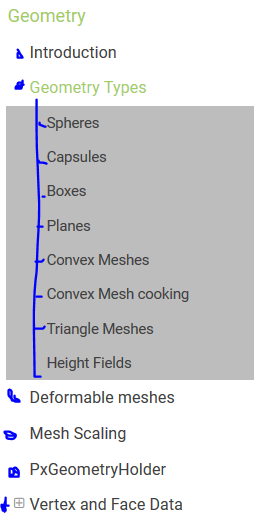## Introduction

This section discusses the PhysX geometry classes. Geometries are used to build shapes for rigid bodies, as collision triggers, and as volumes in PhysX' scene query system. PhysX also provides standalone functions for testing intersection between geometries, raycasting against them, and sweeping one geometry against another.

Geometries are value types, and inherit from a common base class, PxGeometry. Each geometry class defines a volume or surface with a fixed position and orientation. A transform specifies the frame in which the geometry is interpreted. For plane and capsule geometry types PhysX provides helper functions to construct these transforms from common alternative representations.

Geometry类都继承自基类PxGeometry。 每个geometry类都定义了一个具备固定位置和姿态的volume或surface。A transform specifies the frame in which the geometry is interpreted. For plane and capsule geometry types, PhysX provides helper functions to construct these transforms from common alternative representations. (这里的transform指的是啥？位姿坐标么)

Geometries fall into two classes:

germetries可以被划分为两类：

• primitives (PxBoxGeometry, PxSphereGeometry, PxCapsuleGeometry, PxPlaneGeometry) where the geometry object contains all of the data。
primitives类型，(包括PxBoxGeometry, PxSphereGeometry, PxCapsuleGeometry, PxPlaneGeometry）， 该种类型的类对象包含了所有data(相对于下面那种类型不含指针).
• meshes or height fields (PxConvexMeshGeometry, PxTriangleMeshGeometry, PxHeightFieldGeometry), where the geometry object contains a pointer to a much larger object (PxConvexMesh, PxTriangleMesh, PxHeightField respectively) You can use these objects with different scales in each PxGeometry type which references them. The larger objects must be created using a cooking process, described for each type below.
meshes 或 height fields类型（包括PxConvexMeshGeometry, PxTriangleMeshGeometry, PxHeightFieldGeometry），这种类型的类对象包含一个指向一个更大的对象的指针（对应上面括号的三个分别是PxConvexMesh, PxTriangleMesh, PxHeightField）---- 也就是说PxConvexMeshGeometry更高一层，包括了PxConvexMesh。

When passed into and out of the SDK for use as simulation geometry, the geometry is copied into and out of a PxShape class. It can be awkward in this case to retrieve the geometry without knowing its type, so PhysX provides a union-like wrapper class (PxGeometryHolder) that can be used to pass any geometry type by value. Each mesh (or height field) has a reference count that tracks the number of PxShapes whose geometries reference the mesh.

## Geometry Types

### Spheres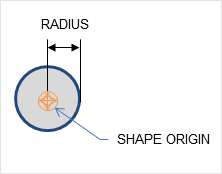A PxSphereGeometry is specified by one attribute, its radius, and is centered at the origin.

### Capsules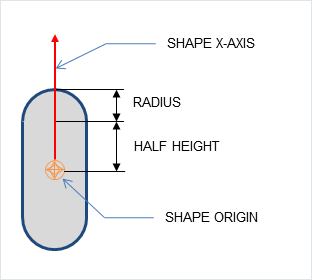A PxCapsuleGeometry is centered at the origin. It is specified by a radius and a half-height value by which its axis extends along the positive and negative X-axis.

To create a dynamic actor whose geometry is a capsule standing upright, the shape needs a relative transform that rotates it around the Z-axis by a quarter-circle. By doing this, the capsule will extend along the Y-axis of the actor instead of the X-axis. Setting up the shape and actor is otherwise the same as for the sphere:

``````PxRigidDynamic* aCapsuleActor = thePhysics->createRigidDynamic(PxTransform(position));

PxTransform relativePose(PxQuat(PxHalfPi, PxVec(0,0,1)));//创建绕z轴旋转90度的四元数

PxShape* aCapsuleShape = PxRigidActorExt::createExclusiveShape(*aCapsuleActor,

aCapsuleShape->setLocalPose(relativePose);//将capsule绕自己的z轴传90度吧，还是绕actor的z轴？？？

PxRigidBodyExt::updateMassAndInertia(*aCapsuleActor, capsuleDensity);

``````

The function PxTransformFromSegment() converts from a line segment defining the capsule axis to a transform and halfheight.

PxTransformFromSegment函数将定义capsule轴的线段转换为 transform和halfheight.

### Boxes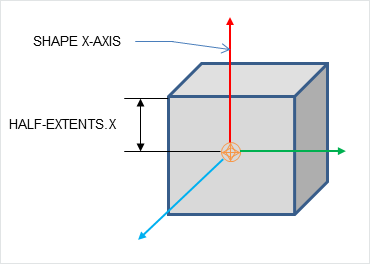A PxBoxGeometry has three attributes, the three extents halved:

``````PxShape* aBoxShape = PxRigidActorExt::createExclusiveShape(*aBoxActor,
PxBoxGeometry(a/2, b/2, c/2), aMaterial);
``````

Where a, b and c are the side lengths of the resulting box.

### Planes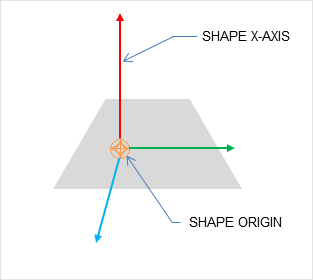Planes divide space into "above" and "below" them. Everything "below" the plane will collide with it.

The Plane lies on the YZ plane with "above" pointing towards positive X. To convert from a plane equation to an equivalent transform（平面方程转换为transform，这里的transform是如何表示一个平面的，用transform类中的那个三维向量表示平面内的一点，用那个四元数表示垂直于该面的法向量吗）, use the function PxTransformFromPlaneEquation(). PxPlaneEquationFromTransform() performs the reverse conversion.

A PxPlaneGeometry has no attributes, since the shape's pose entirely defines the plane's collision volume. (那对于那些有attribute的Geometry类型，其attibute是为了确定collison volume么，例如PxConvexMeshGeometry包括了PxConvexMesh)

Shapes with a PxPlaneGeometry may only be created for static actors.

### Convex Meshes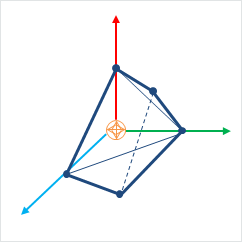A shape is convex if, given any two points within the shape, the shape contains the line between them. A PxConvexMesh is a convex polyhedron represented as a set of vertices and polygonal faces. The number of vertices and faces of a convex mesh in PhysX is limited to 255.

Creating a PxConvexMesh requires cooking. It is assumed here that the cooking library has already been initialized (see Startup and Shutdown.) The following steps explain how to create a simple square pyramid.

First, define the vertices of the convex object:

``````static const PxVec3 convexVerts[] = {PxVec3(0,1,0),PxVec3(1,0,0),PxVec3(-1,0,0),PxVec3(0,0,1),
PxVec3(0,0,-1)};
``````

Then construct a description of the convex data layout:

``````PxConvexMeshDesc convexDesc;
convexDesc.points.count     = 5;
convexDesc.points.stride    = sizeof(PxVec3);
convexDesc.points.data      = convexVerts;
convexDesc.flags            = PxConvexFlag::eCOMPUTE_CONVEX;
``````

Now use the cooking library to construct a PxConvexMesh:

``````PxDefaultMemoryOutputStream buf;
PxConvexMeshCookingResult::Enum result;
if(!cooking.cookConvexMesh(convexDesc, buf, &result))
return NULL;
PxDefaultMemoryInputData input(buf.getData(), buf.getSize());
PxConvexMesh* convexMesh = physics->createConvexMesh(input);
``````

Finally, create a shape using a PxConvexMeshGeometry which instances the mesh:

`PxShape* aConvexShape = PxRigidActorExt::createExclusiveShape(*aConvexActor, `

``````                                                               PxConvexMeshGeometry(convexMesh), aMaterial);
``````

Alternatively the PxConvexMesh can be cooked and directly inserted into PxPhysics without stream serialization. This is useful if real-time cooking is required. It is strongly recommended to use offline cooking and streams. Here is an example showing how to improve cooking speed if needed:

（没有使用PxConvexMeshGeometry

``````PxConvexMeshDesc convexDesc;
convexDesc.points.count     = 5;
convexDesc.points.stride    = sizeof(PxVec3);
convexDesc.points.data      = convexVerts;
convexDesc.flags            = PxConvexFlag::eCOMPUTE_CONVEX | PxConvexFlag::eDISABLE_MESH_VALIDATION |
PxConvexFlag::eFAST_INERTIA_COMPUTATION;

#ifdef _DEBUG
// mesh should be validated before cooking without the mesh cleaning
bool res = theCooking->validateConvexMesh(convexDesc);
PX_ASSERT(res);
#endif

PxConvexMesh* aConvexMesh = theCooking->createConvexMesh(convexDesc,
thePhysics->getPhysicsInsertionCallback()); //红色函数是什么意思。
``````

Please note that mesh validation is required for debug and checked builds, as creating meshes from unvalidated input descriptors may result in undefined behavior. Providing PxConvexFlag::eFAST_INERTIA_COMPUTATION flag the volume integration will use SIMD code path which does faster computation but with lesser precision.

SIMD全称Single Instruction Multiple Data，单指令多数据流，能够复制多个操作数，并把它们打包在大型寄存器的一组指令集。

The user can optionally provide a per-instance PxMeshScale in the PxConvexMeshGeometry. The scale defaults to identity. Negative scale is not supported for convex meshes.

PxConvexMeshGeometry also contains flags to tweak some aspects of the convex object. By default the system computes approximate (loose) bounds around convex objects. Using PxConvexMeshGeometryFlag::eTIGHT_BOUNDS enables smaller/tighter bounds, which are more expensive to compute but could result in improved simulation performance when a lot of convex objects are interacting with each other.

PxConvexMeshGeometry 包含一些flags， 可以用来调整convex。 在默认情况下physx会计算convx的大约的bounds. 用户可以使用PxConvexMeshGeometryFlag::eTIGHT_BOUNDS让physx计算更加精确的bound,这回导致更多的计算，但会在许多objects相互交互的时候，提高仿真效果。

### Convex Mesh cooking

Convex Mesh cooking transforms the mesh data into a form which allows the SDK to perform efficient collision detection. The input to cooking is defined using the input PxConvexMeshDesc.

Convex Mesh cooking将mesh数据转换为一种格式，physx可以使用该中格式做高效的碰撞检测(找到cooking的意义了)！（在上面的例子已经看到）cooking函数的输入需要一个PxConvexMeshDesc。

There are different ways to fill in this structure, depending on whether you want to produce a convex mesh starting from just a cloud of vertices, or whether you have the vertices and faces of a polyhedron already.

If Only Vertex Points are Provided

a 如果用户仅仅提供了顶点数据：

When providing only vertices, set the PxConvexFlag::eCOMPUTE_CONVEX flag to compute the mesh:

``````PxConvexMeshDesc convexDesc;
convexDesc.points.count     = 20;
convexDesc.points.stride    = sizeof(PxVec3);
convexDesc.points.data      = convexVerts;
convexDesc.flags            = PxConvexFlag::eCOMPUTE_CONVEX;
convexDesc.maxVerts         = 10;

PxDefaultMemoryOutputStream buf;
if(!cooking.cookConvexMesh(convexDesc, buf))
return NULL;
``````

The algorithm tries to create a convex mesh from the source vertices. The field convexDesc.vertexLimit specifies the limit for the maximum number of vertices in the resulting hull.

This routine can sometimes fail when the source data is geometrically challenging, for example if it contains a lot of vertices close to each-other. If cooking fails, an error is reported to the error stream and the routine returns false.

If PxConvexFlag::eCHECK_ZERO_AREA_TRIANGLES is used, the algorithm does not include triangles with an area less than PxCookingParams::areaTestEpsilon. If the algorithm cannot find 4 initial vertices without a small triangle, PxConvexMeshCookingResult::eZERO_AREA_TEST_FAILED is returned. This means that the provided vertices were in a very small area and the cooker could not produce a valid hull. The toolkit helper function PxToolkit::createConvexMeshSafe illustrates the most robust strategy for convex mesh cooking. First it tries to create the regular convex hull. If that fails it will use an AABB or OBB.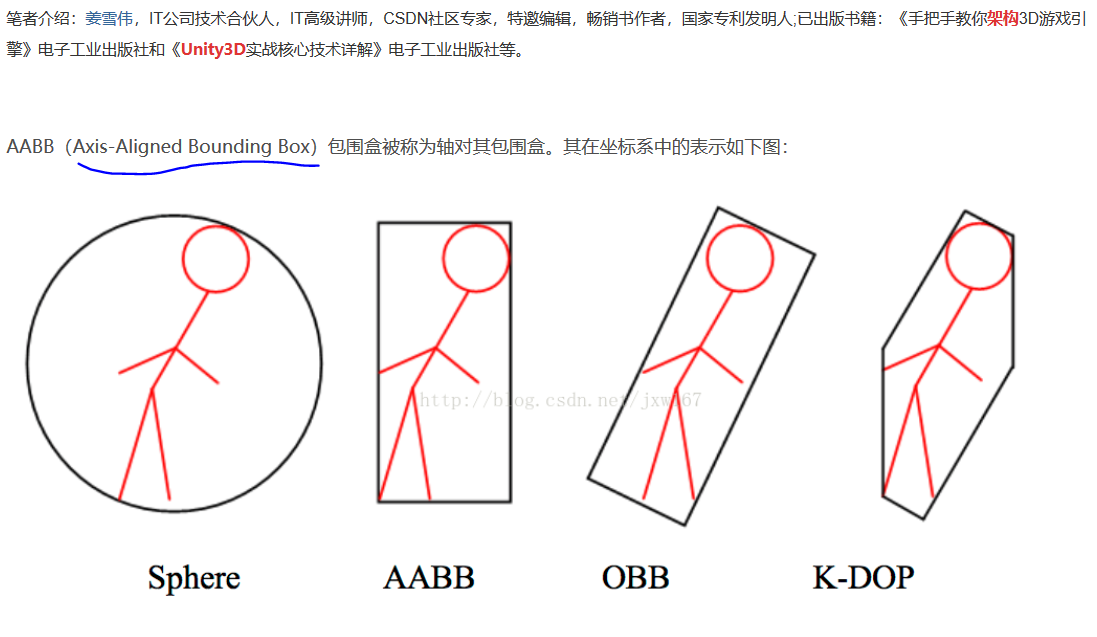It is recommended to provide vertices around origin and put transformation in PxShape, otherwise addional PxConvexFlag::eSHIFT_VERTICES flag for the mesh computation.

If huge amount of input vertices are provided, it might be useful to quantize the input vertices, in this case use PxConvexFlag::eQUANTIZE_INPUT and set the required PxConvexMeshDesc::quantizedCount.

Quickhull Algorithm

This algorithm creates a convex mesh whose vertices are a subset of the original vertices, and the number of vertices is guaranteed to be no more than the specified maximum.

The Quickhull algorithm performs these steps:

• Cleans the vertices - removes duplicates etc.  消除重复的顶点
• Finds a subset of vertices, no more than vertexLimit, that enclose the input set.
• If the vertexLimit is reached, expand the limited hull around the input vertices to ensure we encapsulate all the input vertices.
• Compute a vertex map table. (Requires at least 3 neighbor polygons for each vertex.)
• Checks the polygon data - verifies that all vertices are on or inside the hull, etc.
• Computes mass and inertia tensor assuming density is 1.
• Saves data to stream.  https://www.xiami.com/song/mTxqAp79874

When the hull is constructed each new vertex added must be further than PxCookingParams::planeTolerance from the hull, if not that vertex is dropped.

Vertex Limit Algorithms

If a vertex limit has been provided, there are two algorithms that handle vertex limitation.

The default algorithm computes the full hull, and an OBB around the input vertices. This OBB is then sliced with the hull planes until the vertex limit is reached. The default algorithm requires the vertex limit to be set to at least 8, and typically produces results that are much better quality than are produced by plane shifting.

When plane shifting is enabled (PxConvexFlag::ePLANE_SHIFTING), the hull computation stops when vertex limit is reached. The hull planes are then shifted to contain all input vertices, and the new plane intersection points are then used to generate the final hull with the given vertex limit. Plane shifting may produce sharp edges to vertices very far away from the input cloud, and does not guarantee that all input vertices are inside the resulting hull. However, it can be used with a vertex limit as low as 4, and so may be a better choice for cases such as small pieces of debris with very low vertex counts.

Vertex Points, Indices and Polygons are Provided

b 如果用户提供了Vertex Points, Indices and Polygons

To create a PxConvexMesh given a set of input vertices (convexVerts) and polygons (hullPolygons):

``````PxConvexMeshDesc convexDesc;
convexDesc.points.count             = 12;
convexDesc.points.stride            = sizeof(PxVec3);
convexDesc.points.data              = convexVerts;
convexDescPolygons.polygons.count   = 20;
convexDescPolygons.polygons.stride  = sizeof(PxHullPolygon);
convexDescPolygons.polygons.data    = hullPolygons;
convexDesc.flags                    = 0;

PxDefaultMemoryOutputStream buf;
if(!cooking.cookConvexMesh(convexDesc, buf))
return NULL;
``````

When points and polygons are provided, the SDK validates the mesh and creates the PxConvexmesh directly. This is the fastest way to create a convex mesh. Note that the SDK requires at least 3 neighbor polygons for each vertex. Otherwise acceleration structure for PCM is not created and it does result in performance penalty if PCM is enabled.  ---这样速度最快，要求每个vertex都有对应的至少三个相邻面。

(NOTE: the SDK should reject such a mesh as invalid)

Internal steps during convex cooking:

• Compute vertex map table, requires at least 3 neighbor polygons for each vertex.
• Check polygons data - check if all vertices are on or inside the hull, etc.
• Compute mass and inertia tensor assuming density 1.
• Save data to stream.

### Triangle Meshes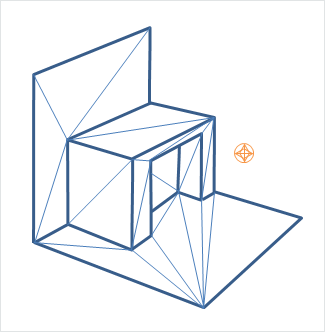Like graphical triangle meshes, a collision triangle mesh consists of a collection of vertices and the triangle indices. Triangle mesh creation requires use of the cooking library. It is assumed here that the cooking library has already been initialized (see Startup and Shutdown.):

collision triangle mesh由一系列的 顶点 和 三角形索引 构成。  创建triangle mesh需要用到cooking library。

``````PxTriangleMeshDesc meshDesc;
meshDesc.points.count           = nbVerts;
meshDesc.points.stride          = sizeof(PxVec3);
meshDesc.points.data            = verts;

meshDesc.triangles.count        = triCount;
meshDesc.triangles.stride       = 3*sizeof(PxU32);
meshDesc.triangles.data         = indices32; //存储的是索引吧，即 某个三角形对应哪三个顶点。

PxDefaultMemoryOutputStream writeBuffer;
PxTriangleMeshCookingResult::Enum result;
bool status = cooking.cookTriangleMesh(meshDesc, writeBuffer,result);
if(!status)
return NULL;

``````

Alternatively PxTriangleMesh can be cooked and directly inserted into PxPhysics without stream serialization. This is useful if real-time cooking is required. It is strongly recommended to use offline cooking and streams. Example how to improve cooking speed if needed:

(与ConvexMesh类似)PxTriangleMesh 可以被cooked并直接插入到PxPhysics中，即跳过stream serialization。 当需要实时cooking时，这是非常有用的。 强烈建议使用offline cooking和stream的方式。 提高cooking speed的例子：

``````PxTolerancesScale scale;
PxCookingParams params(scale);
// disable mesh cleaning - perform mesh validation on development configurations
params.meshPreprocessParams |= PxMeshPreprocessingFlag::eDISABLE_CLEAN_MESH;
// disable edge precompute, edges are set for each triangle, slows contact generation
params.meshPreprocessParams |= PxMeshPreprocessingFlag::eDISABLE_ACTIVE_EDGES_PRECOMPUTE;
// lower hierarchy for internal mesh
params.meshCookingHint = PxMeshCookingHint::eCOOKING_PERFORMANCE;

theCooking->setParams(params);

PxTriangleMeshDesc meshDesc;
meshDesc.points.count           = nbVerts;
meshDesc.points.stride          = sizeof(PxVec3);
meshDesc.points.data            = verts;

meshDesc.triangles.count        = triCount;
meshDesc.triangles.stride       = 3*sizeof(PxU32);
meshDesc.triangles.data         = indices32;

#ifdef _DEBUG
// mesh should be validated before cooked without the mesh cleaning
bool res = theCooking->validateTriangleMesh(meshDesc);
PX_ASSERT(res);
#endif

PxTriangleMesh* aTriangleMesh = theCooking->createTriangleMesh(meshDesc,
thePhysics->getPhysicsInsertionCallback());
``````

Indices can be 16 or 32 bit. The strides used here assume that vertices and indices are arrays of PxVec3s and 32bit integers respectively with no gaps in the data layout.

Returned result enum PxTriangleMeshCookingResult::eLARGE_TRIANGLE does warn the user if the mesh contains large triangles, which should be tessellated to ensure better simulation and CCT stability.

Like height fields, triangle meshes support per-triangle material indices. To use per-triangle materials for a mesh, provide per-triangle indices to the cooking library in the mesh descriptor. Later, when creating the PxShape, supply a table mapping the index values in the mesh to material instances.

Triangle Mesh cooking

Triangle mesh cooking proceeds as follows:

• Check validity of input vertices.
• Weld vertices and check triangle sizes.
• create acceleration structure for queries.
• Compute edge convexity information and adjacencies.
• Save data to stream.

Note that mesh cleaning may result in the set of triangles produced by cooking being a subset different from the original input set. Mesh cleaning removes invalid triangles (containing out-of-range vertex references), duplicate triangles, and zero-area triangles. When this happens, PhysX optionally outputs a mesh remapping table that links each internal triangle to its source triangle in the user's data.

There are multiple parameters to control mesh creation.

In PxTriangleMeshDesc:

• materialIndices defines per triangle materials.  When a triangle mesh collides with another object, a material is required at the collision point. If materialIndices is NULL, then the material of the PxShape instance is used.
PxTriangleMeshDesc结构中的materialIndices成员 定义每个triangle的material索引值。 当triangle mesh与另一个object碰撞时， 碰撞点的trangle的material需要被使用。 如果该成员为NULL,则默认使用PxShape的material.

In PxCookingParams:

• scale defines Tolerance scale is used to check if cooked triangles are not too huge. This check will help with simulation stability.
scale成员定义Tolerance scale ，用于检测triangle是否太大。这种检测有助于仿真稳定性。

• suppressTriangleMeshRemapTable specifies whether the face remap table is created. If not, this saves a significant amount of memory, but the SDK will not be able to provide information about which original mesh triangle is hit in collisions, sweeps or raycasts hits.
suppressTriangleMeshRemapTable 成员指定是否创建face remap table.  如果不创建可以节省很多内存， 但是， 如果不创建的话，当碰撞、sweep、raycast发生时,SDK无法找到哪个original triangle被击中。

• buildTriangleAdjacencies specifies if the triangle adjacency information is created. The adjacent triangles can be retrieved for a given triangle using the getTriangle.

• meshPreprocessParams specifies mesh pre-processing parameters.
meshPreprocessParams 成员指定mesh的预处理参数：

• PxMeshPreprocessingFlag::eWELD_VERTICES enables vertex welding during triangle mesh cooking.

• PxMeshPreprocessingFlag::eDISABLE_CLEAN_MESH disables mesh clean process. Vertices duplicities are not searched, huge triangles test is not done. Vertices welding is not done. Does speed up the cooking.
• PxMeshPreprocessingFlag::eDISABLE_ACTIVE_EDGES_PRECOMPUTE disables vertex edge precomputation. Makes cooking faster but slow up contact generation.
• meshWeldTolerance - If mesh welding is enabled, this controls the distance at which vertices are welded. If mesh welding is not enabled, this value defines the acceptance distance for mesh validation. Provided no two vertices are within this distance, the mesh is considered to be clean. If not, a warning will be emitted. Having a clean mesh is required to achieve the best possible performance.   -- 看起来welding的意思是，如果两个顶点的距离太小，则合并为一个？

• midphaseDesc specifies the desired midphase acceleration structure descriptor.

• PxBVH33MidphaseDesc - PxMeshMidPhase::eBVH33 is the default structure. It was the one used in recent PhysX versions up to PhysX 3.3. It has great performance and is supported on all platforms.
• PxBVH34MidphaseDesc - PxMeshMidPhase::eBVH34 is a revisited implementation introduced in PhysX 3.4. It can be significantly faster both in terms of cooking performance and runtime performance, but it is currently only available on platforms supporting the SSE2 instuction set.

PxBVH33MidphaseDesc params:

• meshCookingHint specifies mesh hierarchy construction preferences. Enables better cooking performance over collision performance, for applications where cooking performance is more important than best quality mesh creation.

PxBVH34MidphaseDesc params:

• numTrisPerLeaf specifies the number of triangles per leaf. Less triangles per leaf produces larger meshes with general better runtime performance and worse cooking performance.

###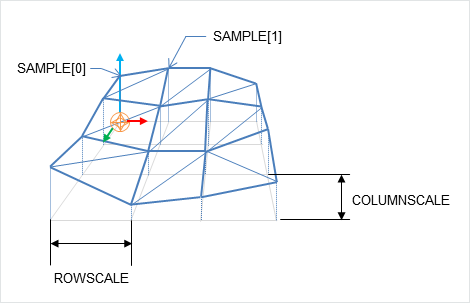Local space axes for the height fields are:

• Row - X axis
• Column - Z axis
• Height - Y axis

As the name suggests, terrains can be described by just the height values on a regular, rectangular sampling grid:

``````PxHeightFieldSample* samples = (PxHeightFieldSample*)alloc(sizeof(PxHeightFieldSample)*
(numRows*numCols));
``````

Each sample consists of a 16 bit integer height value, two materials (for the two triangles in the samples rectangle) and a tessellation flag.

The flag and materials refer to the cell below and to the right of the sample point, and indicate along which diagonal to split it into triangles, and the materials of those triangles. A special predefined material PxHeightFieldMaterial::eHOLE specifies a hole in the height field. See the reference documentation for PxHeightFieldSample for more details.

flag和material的作用：refer to the cell below and to the right of the sample point, 指示沿着哪个方向画斜线以将长方形分成两个三角形，指示三角形的material. 特殊的预定义material： PxHeightFieldMaterial::eHOLE表示一个洞，详见PxHeightFieldSample文档。

Tesselation flag not set Tesselation flag set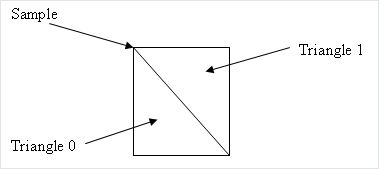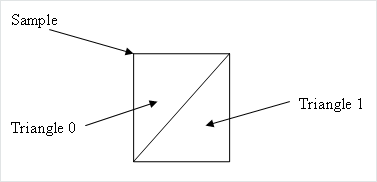Examples:

Tesselation flags Result

0,0,0

0,0,0

0,0,0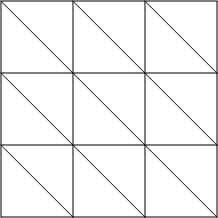1,1,1

1,1,1

1,1,1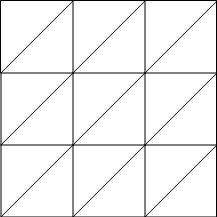1,0,1

0,1,0

1,0,1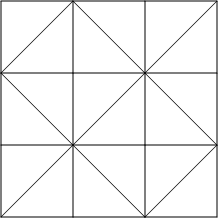To tell the system the number of sampled heights in each direction, use a descriptor to instantiate a PxHeightField object:

``````PxHeightFieldDesc hfDesc;
hfDesc.format             = PxHeightFieldFormat::eS16_TM;
hfDesc.nbColumns          = numCols;
hfDesc.nbRows             = numRows;
hfDesc.samples.data       = samples;
hfDesc.samples.stride     = sizeof(PxHeightFieldSample);

PxHeightField* aHeightField = theCooking->createHeightField(hfDesc,
thePhysics->getPhysicsInsertionCallback());
``````

Now create a PxHeightFieldGeometry and a shape:

``````PxHeightFieldGeometry hfGeom(aHeightField, PxMeshGeometryFlags(), heightScale, rowScale,
colScale);
PxShape* aHeightFieldShape = PxRigidActorExt::createExclusiveShape(*aHeightFieldActor,
hfGeom, aMaterialArray, nbMaterials);
``````

The row and column scales tell the system how far apart the sampled points lie in the associated direction. The height scale scales the integer height values to a floating point range.

The variant of createExclusiveShape() used here specifies an array of materials for the height field, which will be indexed by the material indices of each cell to resolve collisions with that cell. The single-material variant may be used instead, but the height field material indices must all be a single value or the special value eHOLE.

createExclusiveShape函数中的参数aMaterialArray是一个存放material的数组，该数组可以被indexed，heightField的每个cell都有一个material索引值。可以用一个single-material单变量来代替这个数据，这样的话必须保证height field的各个cell的material索引值都相等或者是eHole.

Contact generation with triangle edges at the terrain's borders can be disabled using the PxHeightFieldFlag::eNO_BOUNDARY_EDGES flag, allowing more efficient contact generation when there are multiple heightfield shapes arranged so that their edges touch.

Heightfield cooking

Heightfield data can be cooked in offline and then used to createHeightField. The cooking does precompute and store the edge information. This allows much faster create of the heightfield, since the edges are already precomputed. It is very useful if you need to create heightfields in the runtime, since it does improve the speed of createHeightField significantly.

Heightfield data可以offline地被cook，然后用于创建Height field。 cooking的过程是 pre-compute并存储edge信息，以加速后续的创建过程。这是非常有用的，特别是你想要在运行时创建height field时。

Heightfield cooking proceeds as follows:

Heightfield cooking 的过程：

• Load heightfield samples into internal memory.  加载height field data.
• Precompute edge collision information.          计算edge的碰撞信息。
• Save data to stream.   将计算后的结果保存到stream里。

Heightfields contact generation

The heightfield contact generation approach extracts triangles from the heightfield and utilizes the same low-level contact generation code that is used for triangle meshes. This approach ensures equivalent behavior and performance if triangle meshes or heightfields are used interchangeably. However, with this approach, the heightfield surface has no thickness so fast-moving objects may tunnel if CCD is not enabled.

Height field的contact generation方法： 从heightfield中提取出三角形，使用底层的contact generation code（与triangle mesh使用的方法相同）。这种方法保证了 当heightfield与triangle meshs交替使用时，具有形同的行为和performance.

Heightfields registration

Heightfields are enabled by calling:

``````PxRegisterHeightFields(PxPhysics& physics);
``````

This call must be made before creating any scene, otherwise warnings will be issued. This is a global setting, and it applies to all scenes.

If PxCreatePhysics(...) is called, this will automatically call PxRegisterHeightFields(...). If PxCreateBasePhysics(...) is called, heightfields are not registered by default. If heightfields are used, the application must call the heightfield registration function.

## Deformable meshes  -- 好感兴趣

PhysX supports deformable meshes, i.e. meshes whose vertices move over time (while the topology, i.e. the triangle indices, remains fixed).

Physx支持deformable meshes, 例如， mesh的顶点随着时间变化（拓扑结构不变）。

Deformable meshes are only supported with the PxMeshMidPhase::eBVH33 data structure. Because the mesh vertices are going to be updated, the mapping between the user-defined vertices and PhysX' internal vertices must also be preserved. That is, PhysX should not reorder vertices during cooking. So all cooking operations that could reorder vertices should be disabled, and it is the user's responsability to make sure that the passed vertices are correct w.r.t. disabled operations. For example the mesh cleaning phase should be disabled:

``````cookingParams.midphaseDesc.setToDefault(PxMeshMidPhase::eBVH33);

cookingParams.meshPreprocessParams      = PxMeshPreprocessingFlag::eDISABLE_CLEAN_MESH;``````

It is possible to mix eBVH33 and eBVH34 meshes in the same scene, so the default cooking parameters can still be used for non-deformable / static meshes.

To modify the vertices, use the PxTriangleMesh::getVerticesForModification() and PxTriangleMesh::refitBVH() functions before simulating the scene:

``````// get vertex array
PxVec3* verts = mesh->getVerticesForModification();

// update the vertices here
...

// tell PhysX to update the mesh structure
PxBounds3 newBounds = mesh->refitBVH();
``````

Then use PxScene::resetFiltering() for the corresponding mesh actor, to tell the broadphase its bounds have been modified:

``````scene->resetFiltering(*actor);
``````

When the mesh deforms and moves away from the objects resting on it, said meshes can bounce and jitter slightly on the mesh. Using a slightly negative rest offset for the mesh shape can help reduce this effect:

``````PxShape* shape;
mesh->getShapes(&shape, 1);
shape->setRestOffset(-0.5f);   // something negative, value depends on your world's scale
``````

This will let objects "sink" a bit into the dynamic mesh. That way contacts are not immediately lost and the motion remains smooth. Please refer to the deformable mesh snippet in the SDK for more details.

## Mesh Scaling

A shared PxTriangleMesh or PxConvexMesh may be stretched or compressed when it is instanced by a geometry. This allows multiple instancing of the same mesh with different scale factors applied. Scaling is specified with the PxMeshScale class, which defines scale factors to be applied along 3 orthogonal axes. A factor greater than 1.0 results in stretching, while a factor less than 1.0 results in compression. The directions of the axes are governed by a quaternion, and specified in the local frame of the shape.

Negative mesh scale is supported, with negative values producing a reflection along each corresponding axis. In addition PhysX will flip the normals for mesh triangles when scale.x*scale.y*scale.z < 0.

The following code creates a shape with a PxTriangleMesh scaled by a factor of x along the x-axis, y along the y-axis, and z along the z-axis:

``````// created earlier
PxRigidActor* myActor;
PxTriangleMesh* myTriMesh;
PxMaterial* myMaterial;

// create a shape instancing a triangle mesh at the given scale
PxMeshScale scale(PxVec3(x,y,z), PxQuat(PxIdentity));
PxTriangleMeshGeometry geom(myTriMesh,scale);
PxShape* myTriMeshShape = PxRigidActorExt::createExclusiveShape(*myActor,geom,*myMaterial);
``````

Convex meshes are scaled using the PxMeshScale class in a similar manner. The following code creates a shape with a PxConvexMesh scaled by a factor of x along (sqrt(1/2), 1.0, -sqrt(1/2)), by a factor of y along (0,1,0) and a by a factor of z along (sqrt(1/2), 1.0, sqrt(1/2)):

``````PxMeshScale scale(PxVec3(x,y,z), PxQuat quat(PxPi*0.25f, PxVec3(0,1,0)));
PxConvexMeshGeometry geom(myTriMesh,scale);
PxShape* myConvexMeshShape = PxRigidActorExt::createExclusiveShape(*myActor,geom,*myMaterial);
``````

Height fields can also be scaled, using scale factors stored in PxHeightFieldGeometry. In this case the scale is assumed to be along the axes of the rows, columns and height directions of the height field. The scaling of is demonstrated in SampleNorthPole in SampleNorthPoleBuilder.cpp:

Height field也可被scaled, 使用存储在PxHeightFieldGeometry内部的scale factors。 在这种情况下， 缩放的方向轴是沿着axes of the rows, columns and height directions of the height field。例子在SampleNorthPoleBuilder.cpp:

``````PxHeightFieldGeometry hfGeom(heightField, PxMeshGeometryFlags(), heightScale, hfScale, hfScale);
PxShape* hfShape = PxRigidActorExt::createExclusiveShape(*hfActor, hfGeom, getDefaultMaterial());
``````

In this example, the coordinates along the x and z axes are scaled by hfScale, while the sample heights are scaled by heightScale.

## PxGeometryHolder

When a geometry is provided for a shape, either on creation or with PxShape::setGeometry(), the geometry is copied into the SDK's internal structures. If you know the type of a shape's geometry you may retrieve it directly:

``````PxBoxGeometry boxGeom;
bool status = shape->getBoxGeometry(geometry);
``````

The status return code is set to false if the shape's geometry is not of the expected type.

However, it is often convenient to retrieve a geometry object from a shape without first knowing its type - for example, to call a function which takes a PxGeometry reference as an argument.

PxGeometryHolder is a union-like class that allows the return of a PxGeometry object by value, regardless of type. Its use is illustrated in the createRenderObjectFromShape() function in PhysXSample.cpp:

PxGeometryHolder 是一个类似联合体的类，它运行返回PxGeometry object  by value,而非by type. PhysXSample.cpp中的使用例子：

``````PxGeometryHolder geom = shape->getGeometry();

switch(geom.getType())
{
case PxGeometryType::eSPHERE:
break;
case PxGeometryType::eCAPSULE:
geom.capsule().halfHeight);
break;
...
}
``````

The function PxGeometryHolder::any() returns a reference to a PxGeometry object. For example, to compare two shapes in a scene for overlap:

PxGeometryHolder::any() 返回geometry 实例的引用，例如，以下的例子用于检测同一个scene中的两个shape是否有重叠：

``````bool testForOverlap(const PxShape& s0, const PxShape& s1)
{
return PxGeometryQuery::overlap(s0.getGeometry().any(), PxShapeExt::getGlobalPose(s0),
s1.getGeometry().any(), PxShapeExt::getGlobalPose(s1));
}
``````

## Vertex and Face Data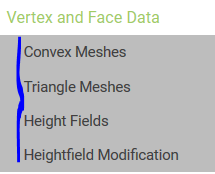Convex meshes, triangle meshes, and height fields can all be queried for vertex and face data. This is particularly useful, for example, when rendering the mesh of the convex shape. The function:

convex mesh, triangle mesh, height field的vertex和face数据可以被query出来。 这一功能特别有用，特别是当渲染mesh的时候。

``````RenderBaseActor* PhysXSample::createRenderObjectFromShape(PxShape* shape, RenderMaterial* material)
``````

in PhysXSample.cpp contains a switch statement with a case for each shape type, illustrating the steps required to query the vertices and faces.

PhysXSample.cpp 中包含一个switch 语句，展示了query vertex和face的步骤。

It is possible to get information about triangle from a triangle mesh or height field using PxMeshQuery::getTriangle function. You can also retrieve adjacent triangle indices for the given triangle (triangle triangleNeighbour[i] shares the edge vertex[i]-vertex[(i+1)%3] with triangle indexed as 'triangleIndex', where vertex is in the range from 0 to 2). To enable this feature the triangle mesh is cooked with buildTriangleAdjacencies parameter set to true.

### Convex Meshes

A convex mesh contains an array of vertices, an array of faces, and an index buffer which concatenates the vertex indices for each face. To unpack a convex mesh, the first step is to extract the shared convex mesh:

convex mesh含有一个存储vertex的数组，一个存储face的数组，一个index buffer，该buffer将face和vertex 索引链接起来。解

``````PxConvexMesh* convexMesh = geom.convexMesh().convexMesh;
``````

Then obtain references to the vertex and index buffers:

``````PxU32 nbVerts = convexMesh->getNbVertices();
const PxVec3* convexVerts = convexMesh->getVertices(); //数组元素是空间点
const PxU8* indexBuffer = convexMesh->getIndexBuffer();//数组元素是unsigned char的数字索引
``````

Now iterate over the array of faces to triangulate them:

``````PxU32 offset = 0;
for(PxU32 i=0;i<nbPolygons;i++) //该convex mesh总共包含nbPolygons个多边形。
{
PxHullPolygon face;
bool status = convexMesh->getPolygonData(i, face);//将第i个多边形取出来存入变量face中
PX_ASSERT(status);

//indexBuffer是一个数组，从数组首部偏移一定的值，对应的是当前face的各个顶点的索引值
const PxU8* faceIndices = indexBuffer + face.mIndexBase;
for(PxU32 j=0;j<face.mNbVerts;j++)//一个face包含mNbVerts个顶点
{
//faceIndices[j]是当前face的第j个顶点的索引值， 利用该索引值从存储顶点的数组找出第j个顶点（空间一个点）
vertices[offset+j] = convexVerts[faceIndices[j]];//找出face的第j个顶点存入vertices顶点数组
//每个顶点都对用一个法向量，属于一个face的各个顶点有相同的法向量:
normals[offset+j] = PxVec3(face.mPlane, face.mPlane, face.mPlane);
}

for(PxU32 j=2;j<face.mNbVerts;j++)//一个face包含mNbVerts个顶点
{
//offset=0,j=2时，以下写入triangles数组的三个值是0，2，1
//offset=0,j=3时，以下写入triangles数组的三个值是0，3，2
//offset=0,j=4时，以下写入triangles数组的三个值是0，4，3
//---所以功能是：从当前face的第0个顶点向各个顶点连线，构成一个个三角形，从而将该face三角化。
*triangles++ = PxU16(offset);//triangles是一个数组，每三个元素一组，对应三个顶点
*triangles++ = PxU16(offset+j);
*triangles++ = PxU16(offset+j-1);
}

offset += face.mNbVerts;//最后，所有face的三角形都被存在triangles数组
}
``````

Observe that the vertex indices of the polygon begin at indexBuffer[face.mIndexBase], and the count of vertices is given by face.mNbVerts.

### Triangle Meshes

Triangle meshes contain arrays of vertices and index triplets which define the triangles by indexing into the vertex buffer. The arrays can be accessed directly from the shared triangle mesh:

Triangle mesh包含一个存储空间顶点的数组，一个存储顶点索引的数组。 可以直接从triangel mesh中取出这些数组：

``````PxTriangleMesh* tm = geom.triangleMesh().triangleMesh;
const PxU32 nbVerts = tm->getNbVertices(); //顶点个数
const PxVec3* verts = tm->getVertices();//存储顶点的数组
const PxU32 nbTris = tm->getNbTriangles(); //三角片的个数
const void* tris = tm->getTriangles(); //存储各个顶点的索引， 老是需要根据索引来找出顶点信息！
``````

The indices may be stored with either 16-bit or 32-bit values, specified when the mesh was originally cooked. To determine the storage format at runtime, use the API call:

``````const bool has16bitIndices = tm->has16BitTriangleIndices();
``````

Assuming that the triangle indices are stored in 16-bit format, find the jth vertex of the ith triangle by:

``````const PxU16* triIndices = (const PxU16*)tris;
const PxU16 index = triIndices[3*i +j];
``````

The corresponding vertex is:

``````const PxVec3& vertex = verts[index];//verts：存储顶点的数组
``````

### Height Fields

The storage of height field data is platform-dependent, and therefore direct access to the height field samples is not provided. Instead, calls are provided to render the samples to a user-supplied buffer.

height field 数据的存储与具体的平台类型相关，因此，不能直接access height field sample(sample的概念？)。 但是， physx提供了函数来render the samples to 用户提供的buffer。

Again, the first step is to retrieve the geometry for the height field:

``````const PxHeightFieldGeometry& geometry = geom.heightField();
``````

The height field has three scaling parameters:(三个缩放系数)

``````const PxReal    rs = geometry.rowScale;
const PxReal    hs = geometry.heightScale;
const PxReal    cs = geometry.columnScale;
``````

And a shared data structure, which stores the row and column count:（取出行数 列数 信息）

``````PxHeightField*  hf = geometry.heightField;
const PxU32     nbCols = hf->getNbColumns();
const PxU32     nbRows = hf->getNbRows();
``````

To render the height field, first extract the samples to an array: (将samples提取到一个数组里)

``````const PxU32 nbVerts = nbRows * nbCols;
PxHeightFieldSample* sampleBuffer = new PxHeightFieldSample[nbVerts]; //定义一个数组。 一个sample就是一个方格么 ？
hf->saveCells(sampleBuffer, nbVerts * sizeof(PxHeightFieldSample));
``````

The samples are stored in row-major order; that is, row0 is stored first, followed by row1, then row2, and so on. Thus the sample corresponding to the ith row and the jth column is i*nbCols + j.

Evaluate the scaled vertices of the height field as follows: 将每个sample都转换成一个顶点？？

``````PxVec3* vertices = new PxVec3[nbVerts];  //上面 const PxU32 nbVerts = nbRows * nbCols;
for(PxU32 i = 0; i < nbRows; i++)
{
for(PxU32 j = 0; j < nbCols; j++)
{
vertices[i * nbCols + j] = PxVec3(PxReal(i) * rs,
PxReal(sampleBuffer[j + (i*nbCols)].height) * hs,
PxReal(j) * cs);
}
}
``````

Then tessellate（网格化） the field from the samples as required.

### Heightfield Modification

Heightfield samples can be modified at runtime in rectangular blocks. In the following code snippet we create a HF and modify its samples:

Height field sample可以在runtime期间被修改in rectangular blocks，例子：创建一个height field并修改它的sample:

`// create a 5x5 HF with height 100 and materials 2,3。 看来每个小方格都有 高度和材料 属性PxHeightFieldSample samples1; //5*5 for (PxU32 i = 0; i < 25; i ++) {     samples1[i].height = 100;     samples1[i].materialIndex0 = 2;//为啥每个sample有两个materialIndex???     samples1[i].materialIndex1 = 3; }PxHeightFieldDesc heightFieldDesc; heightFieldDesc.nbColumns = 5; heightFieldDesc.nbRows = 5; heightFieldDesc.convexEdgeThreshold = 3; heightFieldDesc.samples.data = samples1; heightFieldDesc.samples.stride = sizeof(PxHeightFieldSample);PxPhysics* physics = getPhysics();PxHeightField* pHeightField = cooking->createHeightField(heightFieldDesc, physics->getPhysicsInsertionCallback()); //??//更改其中的10个sample:// create modified HF samples, this 10-sample strip will be used as a modified row // Source samples that are out of range of target heightfield will be clipped with no error.PxHeightFieldSample samplesM; for (PxU32 i = 0; i < 10; i ++) {     samplesM[i].height = 1000;     samplesM[i].materialIndex0 = 1;     samplesM[i].materialIndex1 = 127; }PxHeightFieldDesc desc10Rows; desc10Rows.nbColumns = 1; desc10Rows.nbRows = 10; desc10Rows.samples.data = samplesM; desc10Rows.samples.stride = sizeof(PxHeightFieldSample); pHeightField->modifySamples(1, 0, desc10Rows); // modify row 1 with new sample data`

PhysX does not keep a mapping from the heightfield to heightfield shapes that reference it. Call PxShape::setGeometry on each shape which references the height field, to ensure that internal data structures are updated to reflect the new geometry:
PhysX不会自动地维护 heightfield 与 引用该heightfiel的shape  之间的映射关系。 用户需要对引用了height field的每个shape调用PxShape::setGeometry函数，来保证内部的数据结构被update以reflect the new 修改后的geometry.   //需要进一步理解!

``````PxShape *hfShape = userGetHfShape(); // the user is responsible for keeping track of
// shapes associated with modified HF
hfShape->setGeometry(PxHeightFieldGeometry(pHeightField, ...));
``````

Please also note that PxShape::setGeometry() does not guarantee correct/continuous behavior when objects are resting on top of old or new geometry.

The method PxHeightField::getTimestamp() returns the number of times a heightfield has been modified.

PxHeightField::getTimestamp()函数返回一个height field被修改的次数。